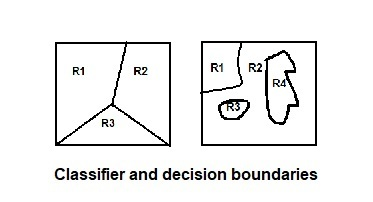Open in App
Not now

# Pattern Recognition | Basics and Design Principles

• Difficulty Level : Easy
• Last Updated : 10 Jan, 2023

Prerequisite – Pattern Recognition | Introduction Pattern Recognition System Pattern is everything around in this digital world. A pattern can either be seen physically or it can be observed mathematically by applying algorithms. In Pattern Recognition, pattern is comprises of the following two fundamental things:

• Collection of observations
• The concept behind the observation
• Differentiate between good and bad features.
• Feature properties.
1. In a statistical-classification problem, a decision boundary is a hypersurface that partitions the underlying vector space into two sets. A decision boundary is the region of a problem space in which the output label of a classifier is ambiguous.Classifier is a hypothesis or discrete-valued function that is used to assign (categorical) class labels to particular data points.
2. Classifier is used to partition the feature space into class-labeled decision regions. While Decision Boundaries are the borders between decision regions.• A Sensor : A sensor is a device used to measure a property, such as pressure, position, temperature, or acceleration, and respond with feedback.
• A Preprocessing Mechanism : Segmentation is used and it is the process of partitioning a data into multiple segments. It can also be defined as the technique of dividing or partitioning an data into parts called segments.
• A Feature Extraction Mechanism : feature extraction starts from an initial set of measured data and builds derived values (features) intended to be informative and non-redundant, facilitating the subsequent learning and generalization steps, and in some cases leading to better human interpretations. It can be manual or automated.
• A Description Algorithm : Pattern recognition algorithms generally aim to provide a reasonable answer for all possible inputs and to perform “most likely” matching of the inputs, taking into account their statistical variation
• A Training Set : Training data is a certain percentage of an overall dataset along with testing set. As a rule, the better the training data, the better the algorithm or classifier performs.
3. Statistical Approach and
4. Structural Approach
5. Descriptive Statistics: It summarizes data from a sample using indexes such as the mean or standard deviation.
6. Inferential Statistics: It draw conclusions from data that are subject to random variation.
7. Sentence Patterns
8. Phrase Patterns
9. Formulas
10. Idioms

Pattern recognition is a subfield of machine learning that focuses on the automatic discovery of patterns and regularities in data. It involves developing algorithms and models that can identify patterns in data and make predictions or decisions based on those patterns.

### There are several basic principles and design considerations that are important in pattern recognition:

1. Feature representation: The way in which the data is represented or encoded is critical for the success of a pattern recognition system. It is important to choose features that are relevant to the problem at hand and that capture the underlying structure of the data.
2. Similarity measure: A similarity measure is used to compare the similarity between two data points. Different similarity measures may be appropriate for different types of data and for different problems.
3. Model selection: There are many different types of models that can be used for pattern recognition, including linear models, nonlinear models, and probabilistic models. It is important to choose a model that is appropriate for the data and the problem at hand.
4. Evaluation: It is important to evaluate the performance of a pattern recognition system using appropriate metrics and datasets. This allows us to compare the performance of different algorithms and models and to choose the best one for the problem at hand.
5. Preprocessing: Preprocessing is the process of preparing the data for analysis. This may involve cleaning the data, scaling the data, or transforming the data in some way to make it more suitable for analysis.
6. Feature selection: Feature selection is the process of selecting a subset of the most relevant features from the data. This can help to improve the performance of the pattern recognition system and to reduce the complexity of the model.

Example:

## Python3

 `from` `collections ``import` `Counter` `def` `predict(fruit):``    ``# Count the number of apples and oranges in the training data``    ``num_apples ``=` `sum``([``1` `for` `f ``in` `training_data ``if` `f[``-``1``] ``=``=` `'apple'``])``    ``num_oranges ``=` `sum``([``1` `for` `f ``in` `training_data ``if` `f[``-``1``] ``=``=` `'orange'``])``    ` `    ``# Find the k nearest neighbors of the fruit``    ``nearest_neighbors ``=` `find_nearest_neighbors(fruit, training_data, k``=``5``)``    ` `    ``# Count the number of apples and oranges among the nearest neighbors``    ``num_apples_nn ``=` `sum``([``1` `for` `nn ``in` `nearest_neighbors ``if` `nn[``-``1``] ``=``=` `'apple'``])``    ``num_oranges_nn ``=` `sum``([``1` `for` `nn ``in` `nearest_neighbors ``if` `nn[``-``1``] ``=``=` `'orange'``])``    ` `    ``# Predict the label of the fruit based on the majority class among the nearest neighbors``    ``if` `num_apples_nn > num_oranges_nn:``        ``return` `'apple'``    ``else``:``        ``return` `'orange'`

Output

` `

My Personal Notes arrow_drop_up